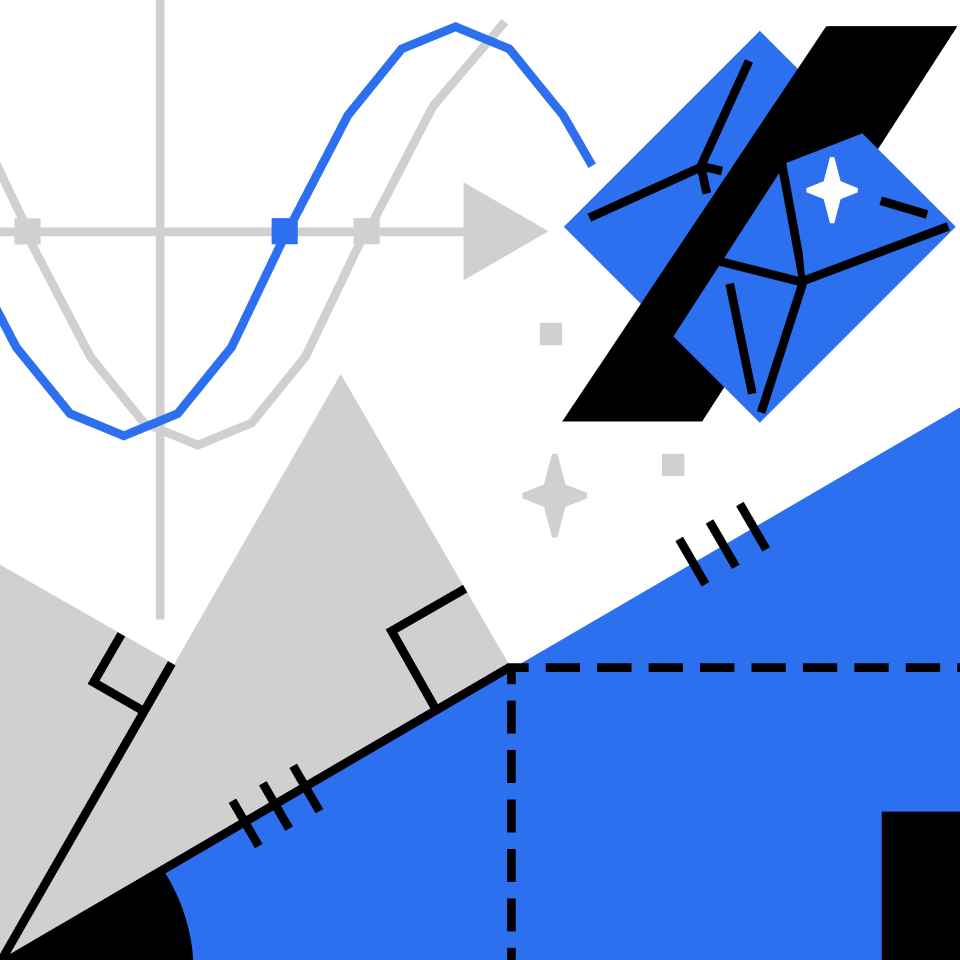## Algebra Puzzles

Flex your skills with some quick and fun algebra puzzles.

Can You Make Sweet Lemonade out of Sour Algebra?

Compare the Weights

What's Triangle + 2 Circles?

Choose the Side!

The Mystery Machine

How Do They Balance?

The Heavier Side

Balancing Shapes

Square Spiral

Circles on the Scale

Efficient Elimination

Painted Cubes

Stabilize These Squares

Solved the Wrong Problem

Series of Squares

Can You Eliminate This Problem?

Difference of Squares Algebra!

The Heaviest Shape

Off Balance

How Many Circles Will Balance a Triangle?

Balanced?

Balance the Triangles

How Many Circles?

Weighty Shapes

When Does The Pattern Fail?

Balance Four Squares

Polygons in the Plane

How Heavy Is the Circle?

Rearrange the Squares

Polygons in a Row

Even Fibonacci

Can a Symmetric Process Lead to an Asymmetric Outcome?

How Much Should You Mix In?

What Comes Next?

Pyramid Scheme

Balance the Shapes

Gracefully Scaling Multiple Dimensions

Is There a Simpler Way?

How Big Is a Half?

Multiplication Magic

Keep the Scales in Balance!

Is There a Simpler Way?

The Heavier Side

Simplification Shortcut

Solve This Shaped Sequence

Sweet Sixteen